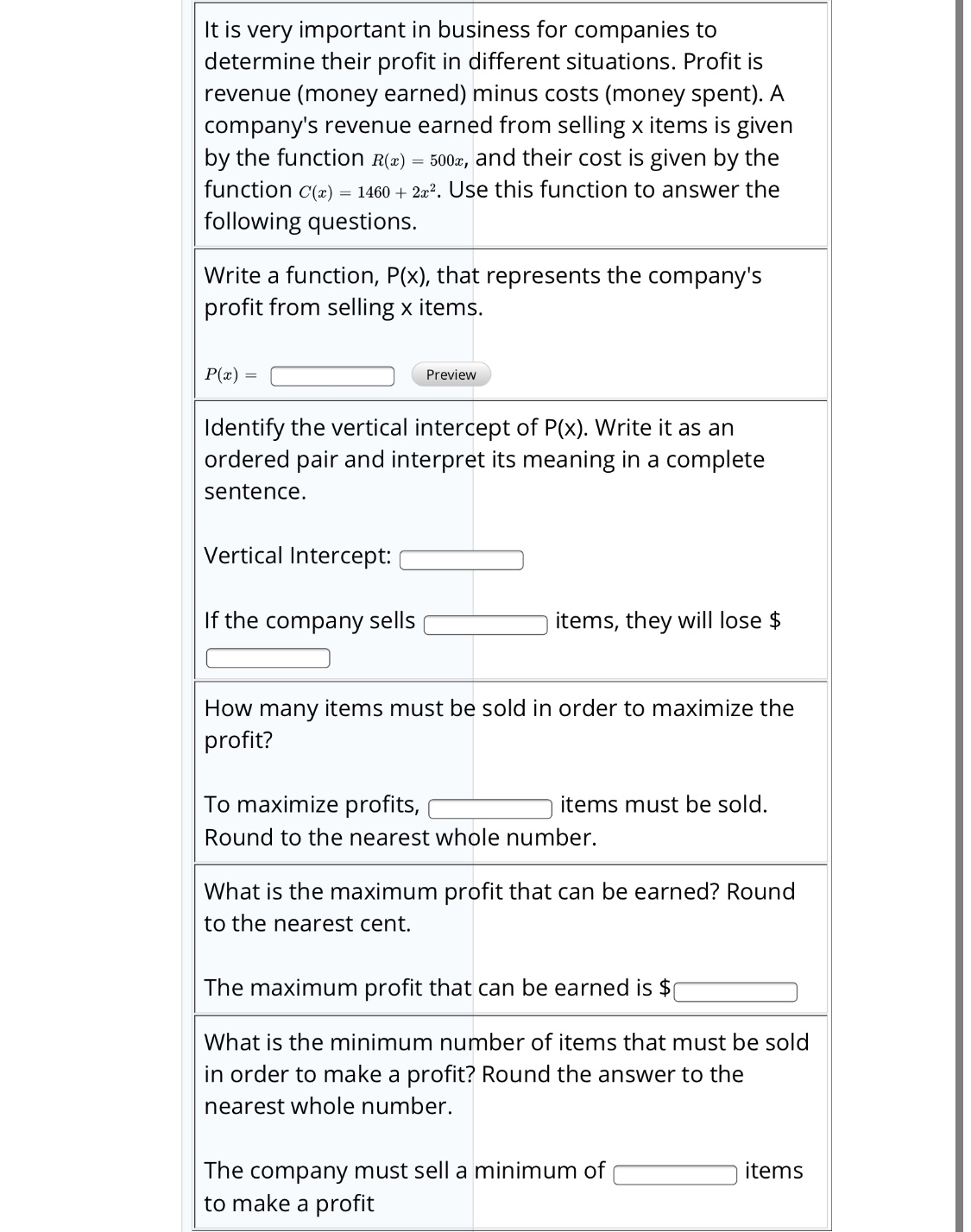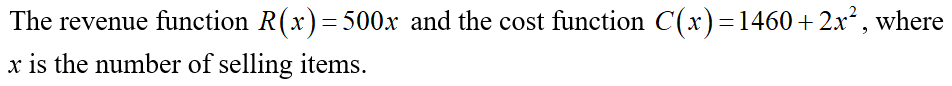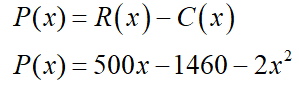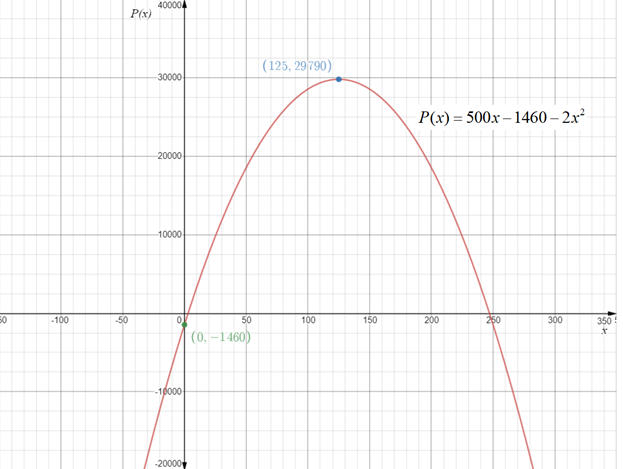# It is very important in business for companies todetermine their profit in different situations. Profit isrevenue (money earned) minus costs (money spent). Acompany's revenue earned from selling x items is givenby the function R(x) =500x, and their cost is given by thefunction c(x) = 1460 + 2a². Use this function to answer thefollowing questions.Write a function, P(x), that represents the company'sprofit from selling x items.P(x)PreviewIdentify the vertical intercept of P(x). Write it as anordered pair and interpret its meaning in a completesentence.Vertical Intercept:If the company sellsitems, they will lose \$How many items must be sold in order to maximize theprofit?To maximize profits,items must be sold.Round to the nearest whole number.What is the maximum profit that can be earned? Roundto the nearest cent.The maximum profit that can be earned is \$What is the minimum number of items that must be soldin order to make a profit? Round the answer to thenearest whole number.The company must sell a minimum ofto make a profititems

Question
1 viewshelp_outlineImage TranscriptioncloseIt is very important in business for companies to determine their profit in different situations. Profit is revenue (money earned) minus costs (money spent). A company's revenue earned from selling x items is given by the function R(x) = 500x, and their cost is given by the function c(x) = 1460 + 2a². Use this function to answer the following questions. Write a function, P(x), that represents the company's profit from selling x items. P(x) Preview Identify the vertical intercept of P(x). Write it as an ordered pair and interpret its meaning in a complete sentence. Vertical Intercept: If the company sells items, they will lose \$ How many items must be sold in order to maximize the profit? To maximize profits, items must be sold. Round to the nearest whole number. What is the maximum profit that can be earned? Round to the nearest cent. The maximum profit that can be earned is \$ What is the minimum number of items that must be sold in order to make a profit? Round the answer to the nearest whole number. The company must sell a minimum of to make a profit items fullscreen
check_circle

Step 1

Since you have posted a question with multiple sub-parts, we will solve first three sub-parts for you. To get remaining sub-part solved please repost the complete question and mention the sub-parts to be solved.

Step 2

We have givenThen the profit function is given by revenue function minus cost function.

i.e.Step 3

Sketch the graph of profit function...

### Want to see the full answer?

See Solution

#### Want to see this answer and more?

Solutions are written by subject experts who are available 24/7. Questions are typically answered within 1 hour.*

See Solution
*Response times may vary by subject and question.
Tagged in